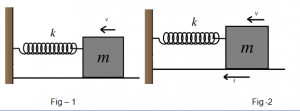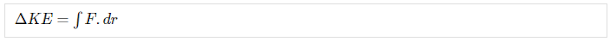Conservative Force and Non-conservative Forces- Definition, Formula, Differences, Videos &amp; FAQs - Padhle.Online
Students' favourite free learning app with LIVE online classes, instant doubt resolution, unlimited practice for classes 6-12, personalized study app for Maths, Science, Social Studies, video e-learning, online tutorial, and more. Download Now!

# Conservative Force and Non-conservative Forces

We know that according to the law of conservation of energy in a closed system, i.e., a system that is isolated from its surroundings, the total energy of the system is conserved”. Conservative force abides by the law of conservation of energy. In this article, let us understand and learn about conservative and non-conservative forces.

## A comparative study between Non-Conservative and Conservative force

In this article let us study in brief about two types of forces namely conservative forces and non-conservative forces. Let us also know the comparison between conservative force and non-conservative force.

Consider the following two situations as shown in the figure.In figure 1, when the mass comes to rest, the spring gets compressed by a distance of ‘x’. The spring then comes to its normal length and mass attains a velocity ‘v’. This compression and elongation continue each time with a maximum value of ‘x’. So we can infer that the total mechanical energy of the system (Block + Spring) remains constant. While in the second case also there will be compression and elongation but the minimum value will keep on decreasing. So we can say that the total mechanical energy of the system is not conserved in this case.

From our observations in the above case, we can now define conservative and non-conservative forces. A conservative force is a force that does zero work done in a closed path. If only these forces act then the mechanical energy of the system remains conserved. Conservative force abides by the law of conservation of energy.

Examples of conservative force: Gravitational force, spring force etc.

On the other hand, non-conservative forces are those forces which cause a loss of mechanical energy from the system. In the above case friction is the non-conservative force. But as we know energy can neither be created nor destroyed hence these forces convert mechanical energy into heat, sound, light etc.

Examples of non-conservative forces are Friction, Air Resistance, and Tension in the cord.

Now, Conservative force has one more property that work done by it is independent of the path taken.

## Work Done By a Conservative Force

Consider the following scenarioWork done by the conservative force in a closed path is zero.

In figure (1) we know work done by the conservative force in a closed path is zero.
W1, A, B + W2, B, A = 0

W1, A, B = – W2, B, A

We also have,

W2, B, A = – W2, A, B

Using the above two equations we get,

W1, A, B = W2, A, B

The above equation shows that work done to move a particle from point A to B through path 1 and 2 as shown in figure 2 will take the same amount of work done. But this statement is not valid for non-conservative forces.

## Difference Between Conservative Force and Non-conservative Force

 Conservative Force Non-Conservative Force W1 = W2 W1 ≠ W2 For closed path: W = 0 For closed path: W ≠ 0 The force is independent of the path. The force depends on the path. Gravitational Force, Spring Force, and Electrostatic force between two electric charges are examples of conservative force. Friction, Air resistance, and Tension in the cord are examples of non-conservative force.

### Conservative force formula

Conservative force is defined as the force such that the work done is independent of the path taken and is dependent only on the initial and final position. Conservative force is applied to the law of conservation of energy. The basic understanding of conservation of energy holds good for conservation of kinetic energy and is given as:$\begin{array}{l}\mathrm{\Delta }KE=\int F.dr\end{array}$

## Frequently Asked Questions – FAQs

True.

### State law of conservation of energy.

The law of conservation of energy states that “In a closed system, i.e., a system that is isolated from its surroundings, the total energy of the system is conserved.”

### Give two examples to explain the law of conservation of energy.

Examples of conservation of energy are:

• In a generator, mechanical energy is converted into electrical energy.
• In a loudspeaker, electrical energy is converted into sound energy.

### What is a non-conservative force?

A non-conservative force is one for which work depends on the path. Hence it is essential to know where the object starts and stops.

### Give some examples for non-conservative forces?

Examples of non-conservative forces are air drag, friction, and tension in the cord.

brings to you the BEST FACULTY for students of Class 9th - 12th. I (Balkishan Agrawal) aim at providing complete preparation for CBSE Board Exams (Maths) along with several other competitive examinations like NTSE, NSO, NSEJS, PRMO, etc. & Maths…